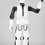# The Ring Game on K[x,y,z]

I recently read about the Ring Game on Mathoverflow http://mathoverflow.net/questions/93276/a-game-on-noetherian-rings, and have been trying to determine winning strategies for each player on various rings. The game has two players and begins with a commutative Noetherian ring R. Player one mods out a nonzero non-unit, and gives the resulting ring to player 2, who repeats the process. The last person to make a legal move (i.e. whoever produces a field) wins. For PIDs the game is trivial: player 1 wins by modding out a single prime element. However, the game becomes far more complicated even in the case of 2-dimensional UFDs. After several days I was unable to determine a winning strategy for either player for Z[x].

I believe the most tractable class of rings are finitely generated commutative algebras over an algebraically closed field K, as for these we can take advantage of the nullstellensatz. So far, I've been able to deal with the cases of K[x] and K[x,y]. Player 1 has a trivial winning strategy for K[x], as it is a PID. For K[x,y], player 1 has a winning strategy as follows:

Player 1 plays x(x+1).

Player 2 plays f(x,y) which vanishes somewhere on V(x(x+1)) but not everywhere (this describes all legal moves). Note that V(f(x,y),x(x+1)) is a finite collection of points possibly union V(x) or V(x+1) but not both. Furthermore, V(f(x,y),x(x+1)) cannot be a single point as the projection of V(f(x,y)) onto the x-axis yields an algebraic set, which must either be a finite collection of points or the entire line and so intersects V(x(x+1)) in at least two points, thus R/(x(x+1),f(x,y)) is not a field.

Player 1 plays ax+by+c which vanishes at exactly one point in V(f(x,y),x(x+1)). This is always possible, since any finite collection of points can be avoided and the lines V(x) or V(x+1) will intersect V(ax+by+c) at most once. The resulting ring is a field, as the ideal I generated by the three plays contains either (ax+by+c,x) or (ax+by+c,x+1), which are maximal, hence is equal to one of these and R/I is a field.


However, I've no idea where to go for K[x,y,z] and beyond. I suspect that K[x,y,z] is a win for player 2, since anything player 1 plays makes the result look vaguely like K[x,y], but on the other hand player 1 could make some pretty nasty plays which might trip up any strategy of player 2's.

So my question is: which player has a winning strategy for K[x,y,z], and what is one such strategy?

Edit: As pointed out in the comments, this winning strategy is wrongNote by Isaac Omolayo
6 years, 1 month ago

This discussion board is a place to discuss our Daily Challenges and the math and science related to those challenges. Explanations are more than just a solution — they should explain the steps and thinking strategies that you used to obtain the solution. Comments should further the discussion of math and science.

When posting on Brilliant:

• Use the emojis to react to an explanation, whether you're congratulating a job well done , or just really confused .
• Ask specific questions about the challenge or the steps in somebody's explanation. Well-posed questions can add a lot to the discussion, but posting "I don't understand!" doesn't help anyone.
• Try to contribute something new to the discussion, whether it is an extension, generalization or other idea related to the challenge.

MarkdownAppears as
*italics* or _italics_ italics
**bold** or __bold__ bold
- bulleted- list
• bulleted
• list
1. numbered2. list
1. numbered
2. list
Note: you must add a full line of space before and after lists for them to show up correctly
paragraph 1paragraph 2

paragraph 1

paragraph 2

[example link](https://brilliant.org)example link
> This is a quote
This is a quote
    # I indented these lines
# 4 spaces, and now they show
# up as a code block.

print "hello world"
# I indented these lines
# 4 spaces, and now they show
# up as a code block.

print "hello world"
MathAppears as
Remember to wrap math in $$ ... $$ or $ ... $ to ensure proper formatting.
2 \times 3 $2 \times 3$
2^{34} $2^{34}$
a_{i-1} $a_{i-1}$
\frac{2}{3} $\frac{2}{3}$
\sqrt{2} $\sqrt{2}$
\sum_{i=1}^3 $\sum_{i=1}^3$
\sin \theta $\sin \theta$
\boxed{123} $\boxed{123}$